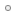1.Established Member
Join Date
Jul 2002
Location
Hastings, UK
Posts
13

##Strange Subtraction problem

Hi all

I have this really anoying problem when trying to do a very simple subtraction, as follows.

The number2 value is always 14, and the number1 value always changes.

Now if the number1 value is lower than 4 then it works fine, but when its higher than 4 say 5 then the miles_val goes into minus e.g -5 when it should obviously be doing this 14 - 5 = 9

Here is the code

number1 = replace(request.querystring("number1"))
number2 = replace(request.querystring("number2"))

if number1 < number2 then
horizontal_num = number2 - number1
miles_val = miles_val + horizontal_num
end if

Any ideas why the number would be jumping to minus when it reaches 10? is it only counting the last 0 digit maybe?Reply With Quote

2.WDF Aficionado
Join Date
Jun 2004
Posts
173
What is the initial value of miles_val and is it reset between calculations?Reply With Quote

####Posting Permissions

• You may not post new threads
• You may not post replies
• You may not post attachments
• You may not edit your posts
•